# Precalculus : Properties of Logarithms

## Example Questions

### Example Question #51 : Properties Of Logarithms

Completely condense the logarithm:.

Possible Answers: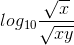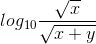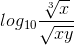None of the other answers.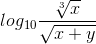Correct answer:Explanation: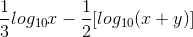Apply the Power property: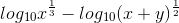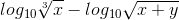Apply the quotient property: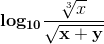### Example Question #52 : Properties Of Logarithms

Simplify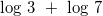Possible Answers: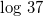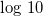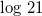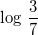Correct answer:Explanation:

By the property of the addition of logarithms with the same base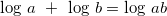As such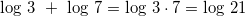### Example Question #53 : Properties Of Logarithms

Condense the following equation: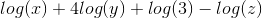Possible Answers: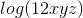Cannot simplify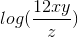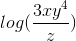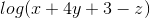Correct answer:Explanation:

Let's use the properties of logarithms to condense this equation.  We will use the follwing three properties

Power Rule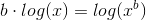Product Rule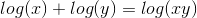Quotient Rule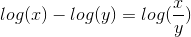Let's first use the power rule to rewrite the second term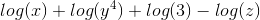Then, we'll use the product rule to combine the first threeterms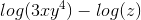Lastly, we'll use the quotient rule to combine into one term### Example Question #54 : Properties Of Logarithms

Express the following in condensed form: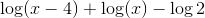Possible Answers: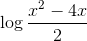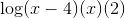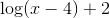Correct answer:Explanation:

To solve, simply remember that when you add logs, you can multiply their insides and when you subtract them, you can divide.

Thus,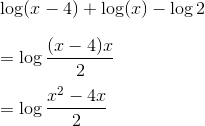### Example Question #55 : Properties Of Logarithms

Express the following in condensed form: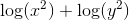Possible Answers: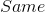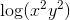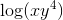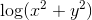Correct answer:Explanation:

To solve, simply remember that when add logs, you multiply their insides. Thus,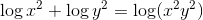### Example Question #56 : Properties Of Logarithms

Condense the logarithm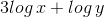Possible Answers: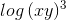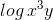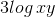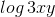Correct answer:Explanation:

In order to condense the logarithmic expression, we use the following properties

•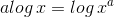•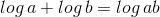As such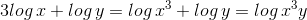### Example Question #57 : Properties Of Logarithms

Expand the following logarithmic expression: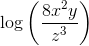Possible Answers: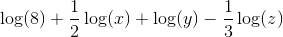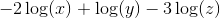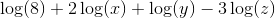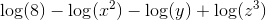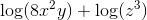Correct answer:Explanation:

We start expanding our logarithm by using the following property: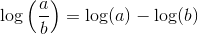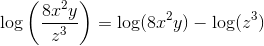Now we have two terms, and we can further expand the first term with the following property: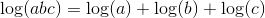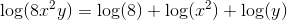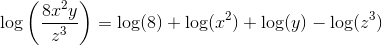Now we only have two logarithms left with nonlinear terms, which we can expand using one final property: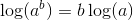Using this property on our two terms with exponents, we obtain the final expanded expression: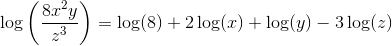### Example Question #58 : Properties Of Logarithms

Expand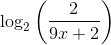.

Possible Answers: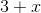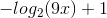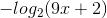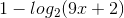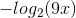Correct answer:Explanation:

To expand, use the quotient property of logs.

The quotient property states: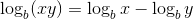Substituting in our given information we get: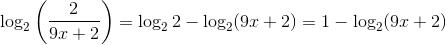### Example Question #59 : Properties Of Logarithms

Which of the following correctly expresses the following logarithm in expanded form?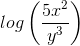Possible Answers: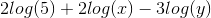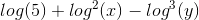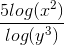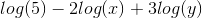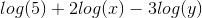Correct answer:Explanation:

Begin by recalling a few logarithm rules:

1) When adding logarithms of like base, multiply the inside.

2) When subtracting logarithms of like base, subtract the inside.

3) When multiplying a logarithm by some number, raise the inside to that power.

Keep these rules in mind as we work backward to solve this problem:Using rule 2), we can get the following: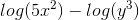Next, use rule 1) on the first part to get: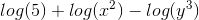Finally, use rule 3) on the second and third parts to get our final answer:### Example Question #60 : Properties Of Logarithms

Express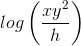in its expanded, simplified form.

Possible Answers: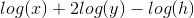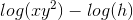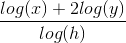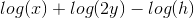Correct answer:Explanation:

Using the properties of logarithms, expand the logrithm one step at a time:

When expanding logarithms, division becomes subtration, multiplication becomes division, and exponents become coefficients.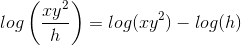.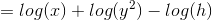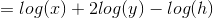### All Precalculus Resources